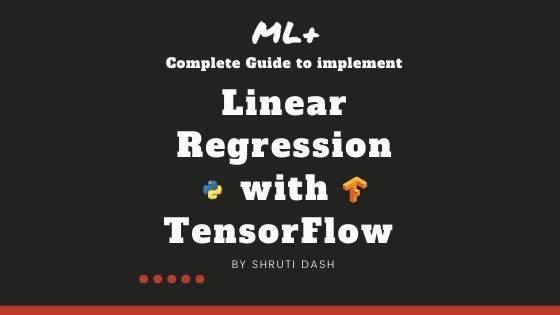## How to implement Linear Regression in TensorFlow

Linear Regression is one of the fundamental machine learning algorithms used to predict a continuous variable using one or more explanatory variables (features). In this tutorial, you will learn how to implement a simple linear regression in Tensorflow 2.0 using the Gradient Tape API. Overview In this tutorial, you will understand: Fundamentals of Linear Regression …